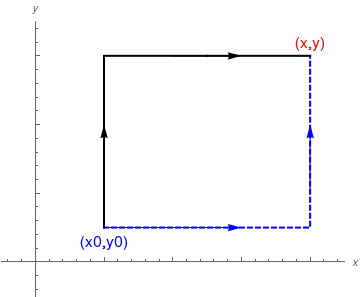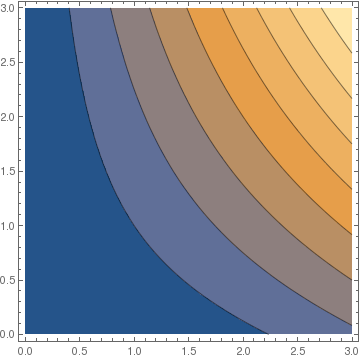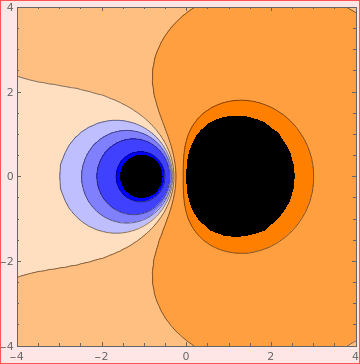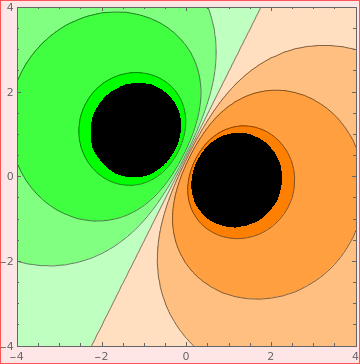# Preface

A differential equation, written in symmetric differential form $$M(x,y)\,{\text d}x + N(x,y)\,{\text d} y =0 ,$$ is exact if and only if there exists a potential function ψ such that its total differential is $${\text d}\psi (x,y) = M(x,y)\,{\text d}x + N(x,y)\,{\text d} y .$$

# Exact Equations

Recall the total differential of a function ψ(x,y) of two variables, denoted by dψ, is given by the expression

${\text d}\psi (x,y) = \frac{\partial \psi}{\partial x} \,{\text d}x + \frac{\partial \psi}{\partial y} \, {\text d}y .$

Let M(x,y) and N(x,y) be two smooth functions having continuous partial derivatives in some domain $$\Omega \subset \mathbb{R}^2$$ without holes. A differential equation, written in differentials

$M(x,y)\,{\text d}x + N(x,y)\, {\text d}y =0$
is called exact if and only if
$\frac{\partial M(x,y)}{\partial y} = \frac{\partial N(x,y)}{\partial x}$
or there exists a smooth function $$\psi (x,y) ,$$ called the potential function, such that its total differential
${\text d}\psi = M(x,y)\,{\text d}x + N(x,y)\, {\text d}y =0 \qquad \mbox{or} \qquad \frac{\partial \psi}{\partial x} = M(x,y), \quad \frac{\partial \psi}{\partial y} = N(x,y).$
A potential function is not unique to which arbitrary constant can be added. Once it is known, the general solution is obtained immediately:
$\psi (x,y) = C , \qquad \mbox{a constant}.$
Since Mathematica uses the command "N" for numerical evaluations, we change notations and use MM(x,y) and NN(x,y) in Mathematica codes instead of M(x,y) and N(x,y), respectively.

There are two approaches to find a potential function corresponding to an exact equation. The first one is based on integration of

$M(x,y) = \frac{\partial \psi}{\partial x} , \qquad N(x,y) = \frac{\partial \psi}{\partial y} \qquad \mbox{or using shortcut}\qquad M = \psi_x , \quad N= \psi_y$
because the total differential of ψ is $${\text d} \psi = \psi_x {\text d}x + \psi_y {\text d} y$$ for all $$x \in \Omega .$$ The second method utilizes line integration, which is preferred for solving initial value problems. Indeed, suppose we are given an initial value problem for an exact equation:
$M(x,y)\,{\text d}x + N(x,y)\, {\text d}y =0 , \qquad y(x_0) = y_0 .$
Let L be arbitrary curve starting at $$(x_0 ,y_0 )$$ and ending at arbitrary point $$(x,y) .$$ This curve or line should be without self-intersections and belong to the domain Ω where functions M(x,y) and N(x,y) possess continuous derivatives. Then the potential function can be obtained as
$\psi (x,y) = \int_L M(x,y)\,{\text d}x + N(x,y)\, {\text d}y .$
In practice, we choose L as a semi-linear line going parallel to axes from initial point $$(x_0 ,y_0 )$$ and finishing at arbitrary point $$(x,y) \in \mathbb{R}^2 .$$ In particular, we can integrate first along vertical axis and then horizontally, or we can integrate horizontally and then vertically.Two contours of integrations along axeses. line1 = Line[{{1, 0.5}, {4, 0.5}, {4, 3}}]; line2 = Line[{{1, 0.5}, {1, 3}, {4, 3}}]; a = {Graphics[{Thick, Dashed, Blue, line1}], Graphics[{Thick, line2}]} b = Graphics[Text[Style["(x,y)", FontSize -> 14, Red], {4.0, 3.2}]] b0 = Graphics[ Text[Style["(x0,y0)", FontSize -> 14, Blue], {1.0, 0.3}]] aa1 = Graphics[Arrow[{{1, 1}, {1, 2}}]] aa2 = Graphics[Arrow[{{2, 3}, {3, 3}}]] aa3 = Graphics[{Blue, Arrow[{{1, 0.5}, {3, 0.5}}]}] aa4 = Graphics[{Blue, Arrow[{{4, 1}, {4, 2}}]}] Show[aa1, aa2, aa3, aa4, a, b, b0, Axes -> True, AxesOrigin -> {0, 0}, PlotRange -> {{-0.5, 4.5}, {-0.5, 3.5}}, AxesLabel -> {x, y}, TicksStyle -> Directive[FontOpacity -> 0, FontSize -> 0]] Two contours of integration. Mathematica code

If we integrate along black line (vertically where dx = 0 and then horizontally where dy = 0), we get

$\psi (x,y) = \int_{y_0}^y N(x_0 ,y)\, {\text d}y + \int_{x_0}^x M(x ,y)\, {\text d}x .$

Now if we integrate along blue dashed line (horizontally where dy = 0 and then vertically where dx = 0), we get

$\psi (x,y) = \int_{x_0}^x M(x ,y_0 )\,{\text d}x + \int_{y_0}^y N(x ,y)\, {\text d}y .$

Example: The equation $$y \,\text{d}x + x \,\text{d}y =0$$ is exact because $$M_y =1 = N_x$$ for $$M= y \quad\mbox{and} \quad N= x .$$ Suppose that the initial condition $$y(2)=3$$ is given.

We type in Mathematica:

MM[x_, y_] = y; NN[x_, y_] = x;
{p1, p2} = {2, 3};
Simplify[D[MM[x, y], y] == D[NN[x, y], x]]
Out = True
psi[X_, Y_] =
Integrate[MM[x, p2], {x, p1, X}] + Integrate[NN[X, y], {y, p2, Y}]
Out= 3 (-2 + X) + X (-3 + Y)

The solution is psi[x,y]==0:

grad[potential_, vars_List] := Map[Function[t, D[potential, t]], vars]

and then check our potential function:

Out= {y,x}
We check the answer with (blue) line integration:
$\psi (x,y) = \int_{2}^x 3\,{\text d}x + \int_{3}^y x\, {\text d}y = 3(x-2) + x( y-3) = xy-6.$
Therefore, the solution becomes ψ =0 or $$xy -6 =0 .$$

Example: Consider the differential equation

$\left( x+ 2y \right) {\text d}x + \left( 2x-3y \right) {\text d}y =0.$
First, we check whether the given equation is exact.
MM=x+2 y; NN=2 x-3 y;
a=TrueQ[D[MM,y]==D[NN,x]];
If[a==True, Print["The equation is exact"], Print["The equation is not exact"]]
Out = The equation is exact
F = Integrate[MM,x]
Out = x^2/2 + 2 x y
derfy = D[F, y] - NN
Out = 3 y
f[y] = Integrate[derfy, y]
Out = (3 y^2)/2
psi = F + f[y]
Out = x^2/2 + 2 x y + (3 y^2)/2
Print["psi=", psi];
Out = psi=x^2/2+2 x y+(3 y^2)/2
Print["Trajectories of psi..."]
Out = Trajectories of psi...
ContourPlot[F,{x,0,3},{y,0,3}]Example: An electrostatic potential built from a collection of point charges qi at positions ii

ElectroStaticPotential[q_, p_, r_] := Sum[q[[i]]/(Norm[r - p[[i]]]), {i, Length[q]}]
ElectroStaticPotential[{Subscript[q, 1], Subscript[q, 2]}, {{Subscript[x, 1], Subscript[y, 1]}, {Subscript[x, 2], Subscript[y, 2]}}, {x, y}] // TraditionalForm
$$\displaystyle \frac{q_1}{\sqrt{| x - x_1 |^2 + |y - y_1 |^2}} + \frac{q_2}{\sqrt{| x - x_2 |^2 + |y - y_2 |^2}}$$
We organize the backgrownd of charge colors, using blue for negative and orange for positive:
c = Join[Table[Lighter[Blue, i/4], {i, 0, 3}], Table[Lighter[Orange, i/4], {i, 3, 0, -1}]];Two charges q1 = -1 and q2 = 2 ContourPlot[ Evaluate[ElectroStaticPotential[{-1, 2}, {{-1, 0}, {1, 0}}, {x, y}]], {x, -4, 4}, {y, -4, 4}, Contours -> {-0.75, -0.25, -0.1, 0, 0.1, 0.25, 0.75}, PlotRange -> 1, ClippingStyle -> Automatix, ContourShading -> c] Electrostatic potential of two charges. Mathematica codeThree charges q1 = -1 and q2 = -1, and q3 = 2 ContourPlot[ Evaluate[ElectroStaticPotential[{-1, -1, 2}, {{-1, 1}, {-1, 1}, {1, 0}}, {x, y}]], {x, -4, 4}, {y, -4, 4}, Contours -> {-0.75, -0.25, -0.1, 0, 0.1, 0.25, 0.75}, PlotRange -> 1, ClippingStyle -> Automatix, ContourShading -> c] Electrostatic potential of three charges. Mathematica code

■